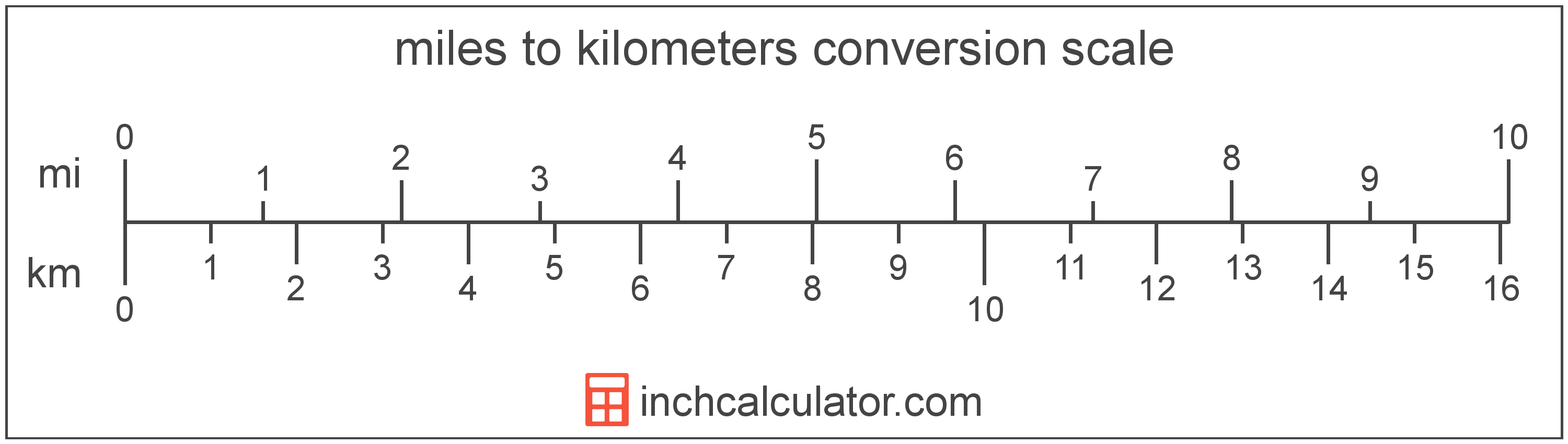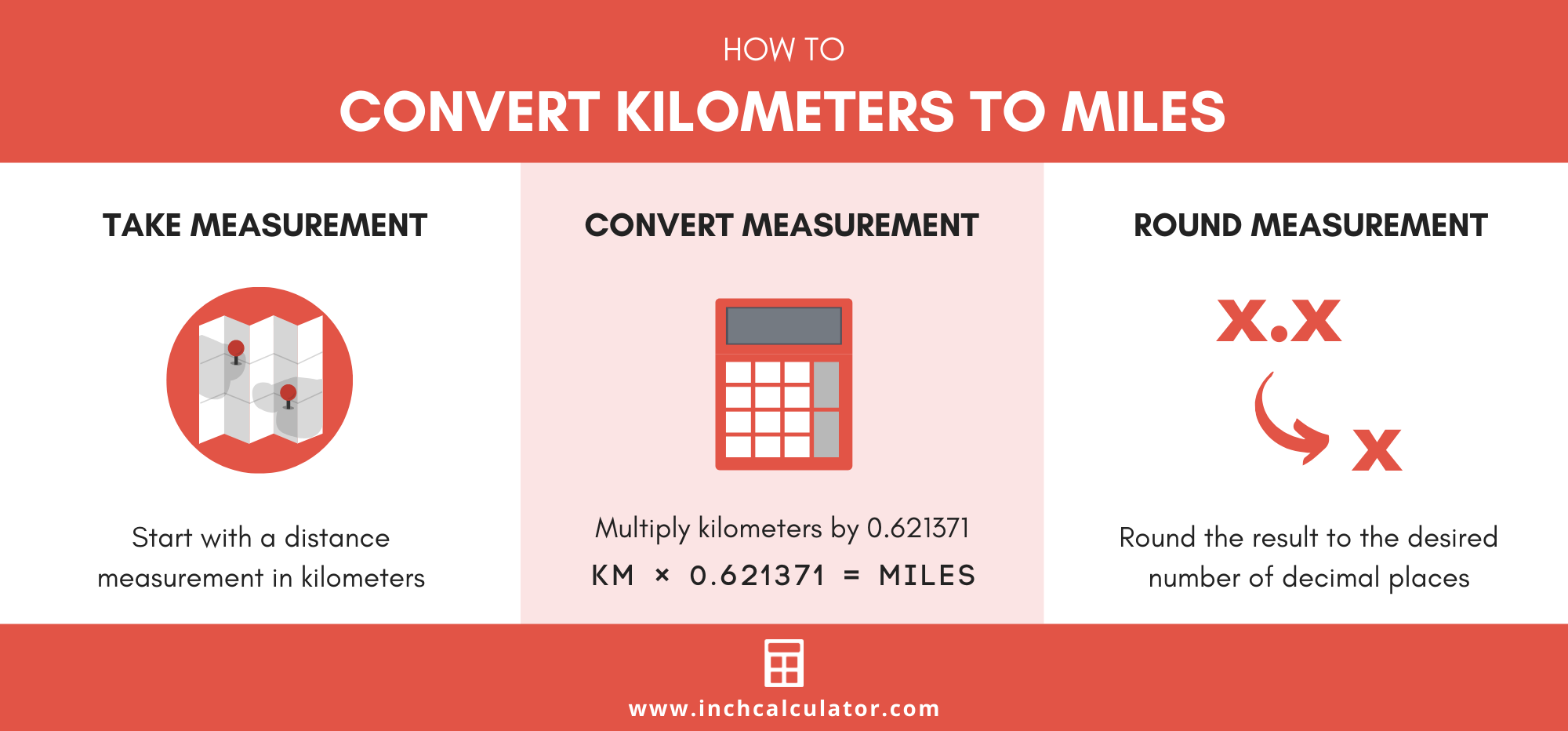# Km To Miles Converter (Kilometers To Miles)

Enter the length in kilometers below to get the value converted to miles.

Results in Miles:1 km = 0.621371 mi

Do you want to convert miles to kilometers?

## How to Convert Kilometers to Miles

The fastest and easiest way to convert km (kilometers) to miles is use this simple formula:

miles = kilometers × 0.621371

The distance in miles is equal to the distance in kilometers multiplied by 0.621371. Since one kilometer is equal to 0.621371 miles, that's the conversion ratio used in the formula.

For example, here's how to convert 10 kilometers to miles using the formula above.

miles = (10 km × 0.621371) = 6.21371 mi

Thus, if you're running a 10k, the distance is actually 6.21371 miles.### How Many Miles Are in a Kilometer?

There are 0.621371 miles in a kilometer, which is why we use this value in the formula above.

1 km = 0.621371 mi

## How to Convert Kilometers to Miles Without a Calculator

Multiplying by the conversion ratio of 0.621371 might be easy with a calculator in hand, but what if you want to convert kilometers to miles in your head?

While it's not precisely accurate, there's actually a pretty straightforward method for converting without a calculator that will get you pretty close.

Follow these three steps to convert kilometers to miles:

• Divide km by 2
• Divide km by 10
• Add these two values together

For example, here's how to convert 50 kilometers to miles using this method.

• 50 km ÷ 2 = 25
• 50 km ÷ 10 = 5
• 25 + 5 = 30 mi

So, that's a pretty quick shortcut to converting to miles in your head without a calculator. And while it's not precisely accurate, it's pretty close to the actual value in miles, which is 31.07.## What Is a Kilometer?

One kilometer is equal to 1,000 meters, which are defined as the distance light travels in a vacuum in 1/299,792,458 of a second. One kilometer is equal to 0.621371 miles.

The kilometer, or kilometre, is a multiple of the meter, which is the SI base unit for length. In the metric system, "kilo" is the prefix for thousands, or 103. Kilometers can be abbreviated as km; for example, 1 kilometer can be written as 1 km.

## What Is a Mile?

The mile is a linear measurement of length equal to exactly 1,609.344 meters or 1.60934 kilometers. One mile is also equal to 5,280 feet or 1,760 yards.

The mile is a US customary and imperial unit of length. Miles can be abbreviated as mi, and are also sometimes abbreviated as m. For example, 1 mile can be written as 1 mi or 1 m.

## Kilometer to Mile Conversion Table

Table showing various kilometer measurements converted to miles.
Kilometers Miles
1 km 0.621371 mi
2 km 1.2427 mi
3 km 1.8641 mi
4 km 2.4855 mi
5 km 3.1069 mi
6 km 3.7282 mi
7 km 4.3496 mi
8 km 4.971 mi
9 km 5.5923 mi
10 km 6.2137 mi
11 km 6.8351 mi
12 km 7.4565 mi
13 km 8.0778 mi
14 km 8.6992 mi
15 km 9.3206 mi
16 km 9.9419 mi
17 km 10.56 mi
18 km 11.18 mi
19 km 11.81 mi
20 km 12.43 mi
21 km 13.05 mi
22 km 13.67 mi
23 km 14.29 mi
24 km 14.91 mi
25 km 15.53 mi
26 km 16.16 mi
27 km 16.78 mi
28 km 17.4 mi
29 km 18.02 mi
30 km 18.64 mi
31 km 19.26 mi
32 km 19.88 mi
33 km 20.51 mi
34 km 21.13 mi
35 km 21.75 mi
36 km 22.37 mi
37 km 22.99 mi
38 km 23.61 mi
39 km 24.23 mi
40 km 24.85 mi

## References

1. Wisconsin Department of Transportation, 2019 Standard Specifications, https://wisconsindot.gov/rdwy/stndspec/ss-99-30.pdf
2. Ambler Thompson and Barry N. Taylor, Guide for the Use of the International System of Units (SI), National Institute of Standards and Technology, https://physics.nist.gov/cuu/pdf/sp811.pdf